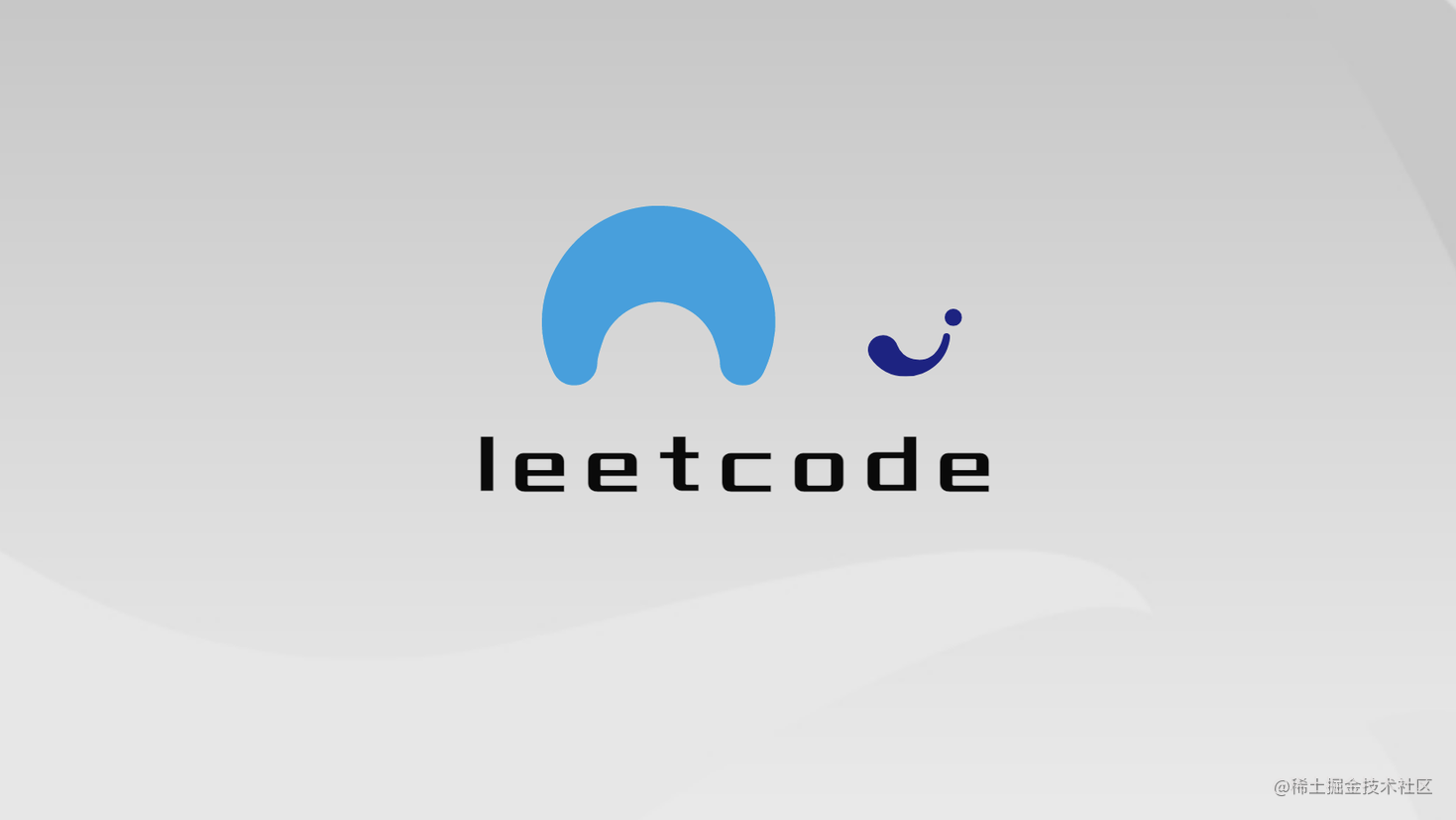# leetcode每日一题系列-反转字符串中的元音字母-「双指针」# leetcode-345-反转字符串中的元音字母

## [题目描述]

`````` 输入："hello"

`````` 输入："leetcode"

• 元音字母不包含字母 "y" 。

Related Topics

• 双指针
• 字符串
• 👍 182 👎 0

leetcode题目链接

## [思路介绍]

### 思路一：双指针

• 暴力法是可解 后续看看时间来不来得及补充一下
• 双指针 l,r 分别指向左右第一个元音字母的索引
• 跳出条件是l指向的索引是否小于r指向的索引
• 每遍历到一个元音字母
• 交换 l r
• l 右移至下一个元音字母索引位置
• r 左移至下一个原因字母索引位置
• 因为java语言限制，String类型不可变，无法直接修改字符串
• 所以空间上需要选择charArray这种类似的数据结构做交换
• 定义寻址函数findl findr
• 定义交换函数swap
• 定义check函数判断是否是原因字母check
• 第一次提交忘记了大小写的问题
``````public String reverseVowels(String s) {
char[] res = s.toCharArray();
int l = findl(res, 0), r = findr(res, s.length() - 1);
while (l < r) {
swap(res, l, r);
l = findl(res, l + 1);
r = findr(res, r - 1);
}
return String.valueOf(res);
}

public int findl(char[] chars, int l) {
while (l < chars.length) {
if (check(chars[l])) {
return l;
}
l++;
}
return l;
}

public int findr(char[] chars, int r) {
while (r >= 0) {
if (check(chars[r])) {
return r;
}
r--;
}
return r;
}

public void swap(char[] chars, int l, int r) {
char temp = chars[l];
chars[l] = chars[r];
chars[r] = temp;
}

public boolean check(char c) {
//大小写
c = Character.toLowerCase(c);
return c == 'a' || c == 'e' || c == 'i' || c == 'o' || c == 'u';
}

• 时间复杂度O(n)
• 空间复杂度O(n)-某些语言允许直接更新字符串的可以优化到O(1)